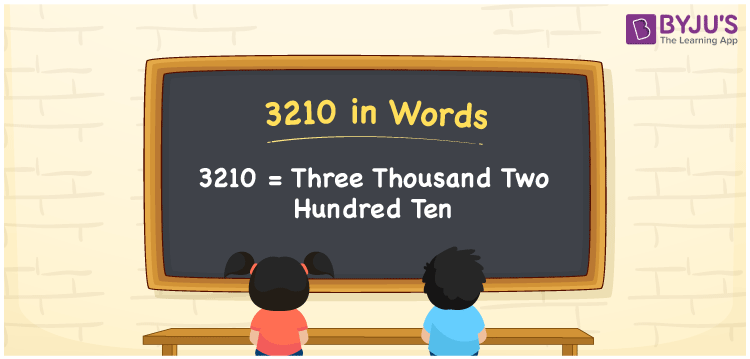# 3210 in Words

3210 in words is written as Three thousand two hundred ten. In both the International System of Numerals and the Indian System of Numerals, 3210 is written as Three thousand two hundred ten. The number 3210 is a Cardinal Number as it represents some quantity. For example, “that flower vase costs 3210 rupees”.

 3210 in Words Three thousand two hundred ten Three thousand two hundred ten in Number 3210

## 3210 in English Words

3210 in English words is read as “Three thousand two hundred ten”.## How to Write 3210 in Words?

To write 3210 in words, we shall use the place value chart. In the place value chart, put 3 in the thousands, 2 in the hundreds, 1 in the tens, and 0 in the ones, respectively. Let us make a place value chart to write the number 3210 in words.

 Thousands Hundreds Tens Ones 3 2 1 0

Thus, we can write the expanded form as

3 × Thousand + 2 × Hundred + 1 × Ten + 0 × One

= 3 × 1000 + 2 × 100 + 1 × 10 + 0 × 1

= 3000 + 200 + 10 + 0

= 3210

= Three thousand two hundred ten.

3210 is a natural number that is the successor of 3209 and the predecessor of 3211.

3210 in words – Three thousand two hundred ten

• Is 3210 an odd number? – No
• Is 3210 an even number? – Yes
• Is 3210 a perfect square number? – No
• Is 3210 a perfect cube number? – No
• Is 3210 a prime number? – No
• Is 3210 a composite number? – Yes

## Frequently Asked Questions on 3210 in Words

Q1

### How to write 3210 in words?

3210 in words is written as Three thousand two hundred ten.
Q2

### How to write 3210 in the International and Indian System of Numerals?

In both, the system of numerals, 3210 in words, is written as Three thousand two hundred ten.
Q3

### How to write 3210 in a place value chart?

In the place value chart, write 3 in the thousands, 2 in the hundreds, 1 in the tens, and 0 in the ones, respectively.